Examples

Chapter 4 Class 11 Mathematical Induction
Serial order wise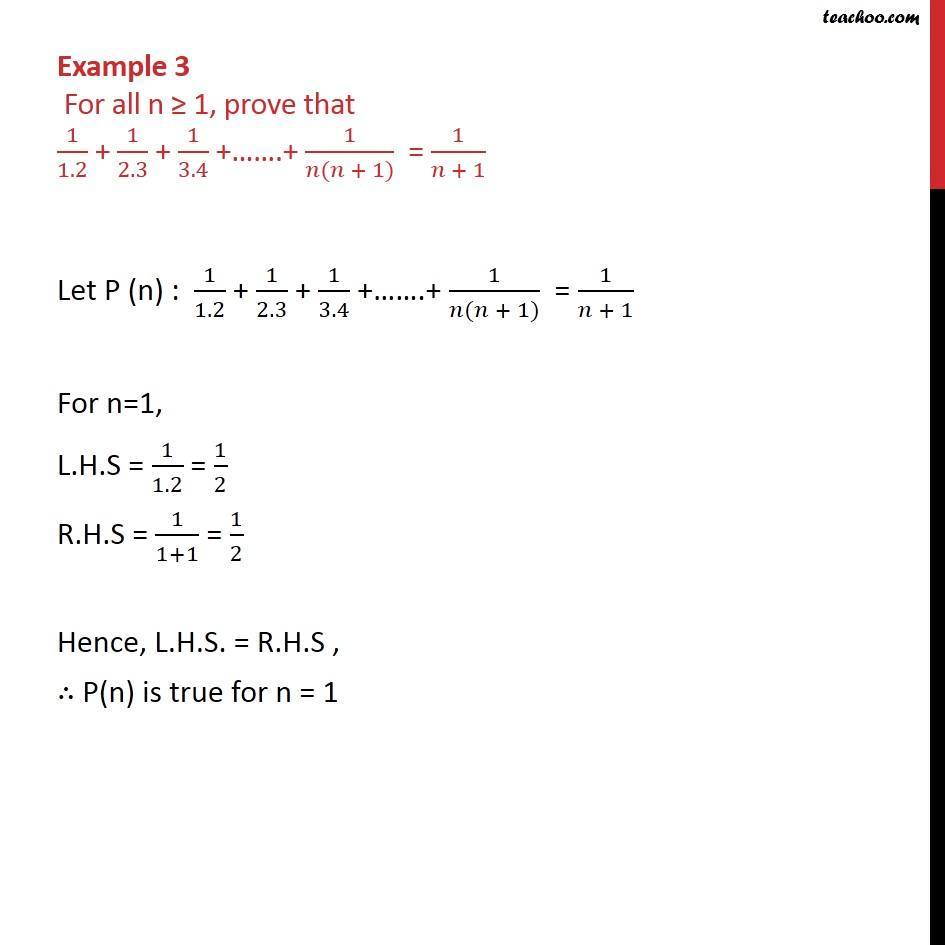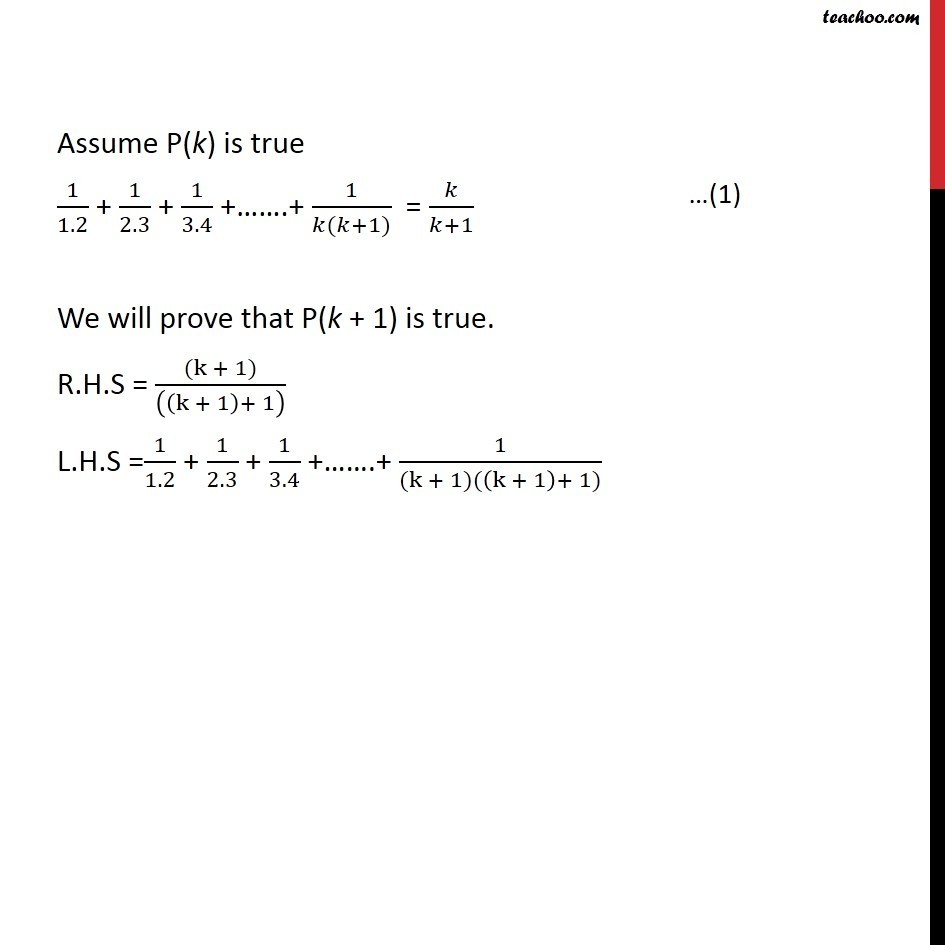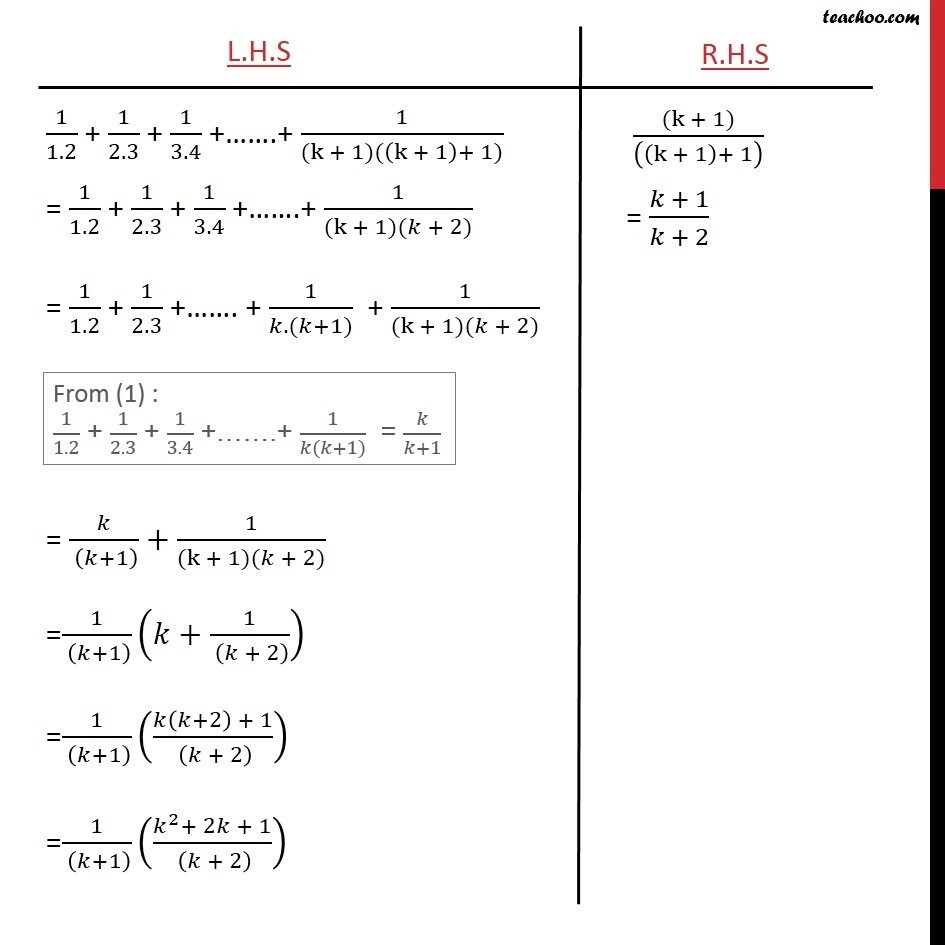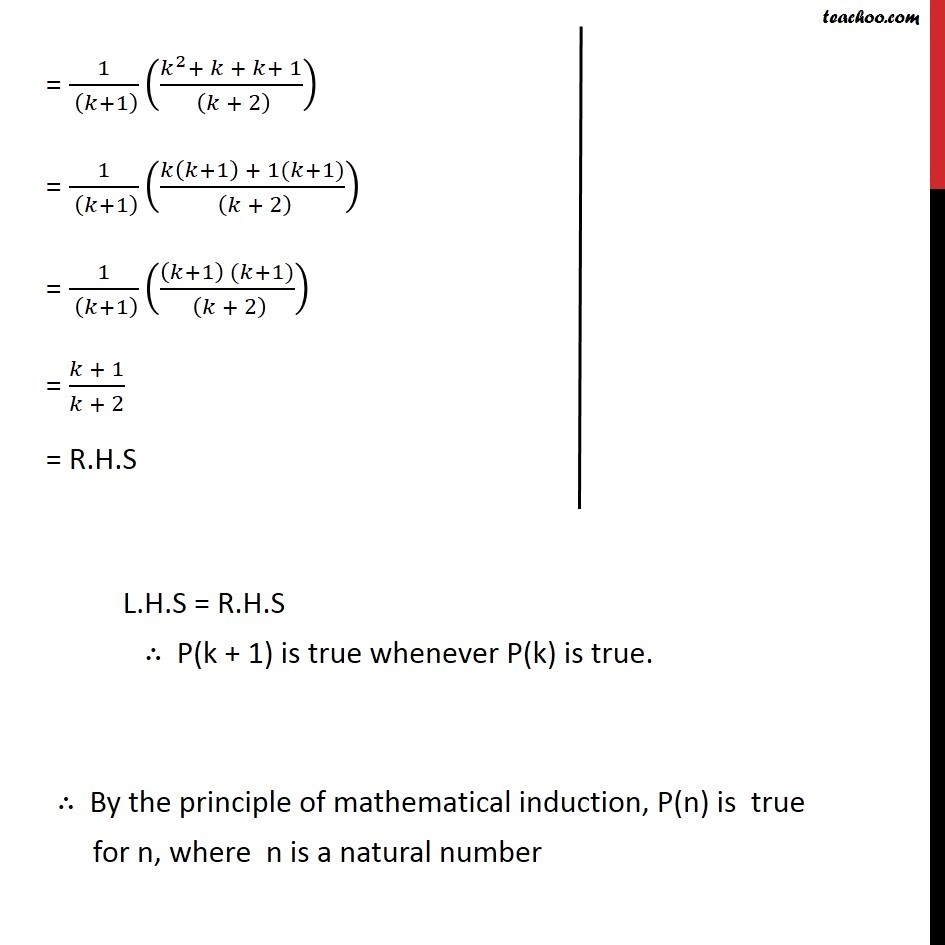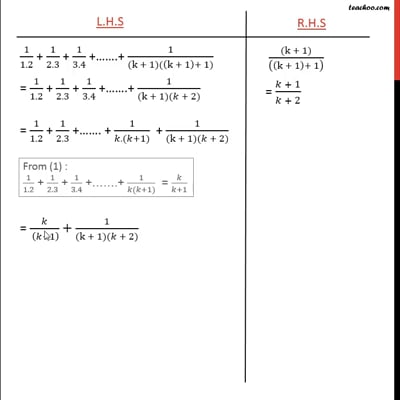This video is only available for Teachoo black users

Get live Maths 1-on-1 Classs - Class 6 to 12

### Transcript

Example 3 For all n ≥ 1, prove that 1/1.2 + 1/2.3 + 1/3.4 +…….+ 1/(𝑛(𝑛 + 1)) = 1/(𝑛 + 1) Let P (n) : 1/1.2 + 1/2.3 + 1/3.4 +…….+ 1/(𝑛(𝑛 + 1)) = 1/(𝑛 + 1) For n=1, L.H.S = 1/1.2 = 1/2 R.H.S = 1/(1+1) = 1/2 Hence, L.H.S. = R.H.S , ∴ P(n) is true for n = 1 Assume P(k) is true 1/1.2 + 1/2.3 + 1/3.4 +…….+ 1/(𝑘(𝑘+1)) = 𝑘/(𝑘+1) We will prove that P(k + 1) is true. R.H.S = ((k + 1))/(((k + 1)+ 1) ) L.H.S =1/1.2 + 1/2.3 + 1/3.4 +…….+ 1/((k + 1)((k + 1)+ 1)) ∴ By the principle of mathematical induction, P(n) is true for n, where n is a natural number